# ComplexBash: A touch of vectors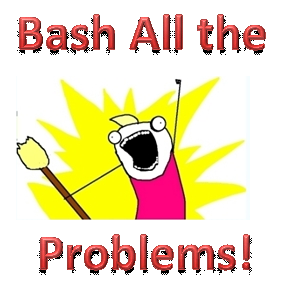This note is related to the previous note, please have a look if you haven't read that one.

This note deals with $$\color{Brown}{\text{the basics of complex numbers}}$$ and $$\color{Purple}{\text{some useful results}}$$ that will be needed in bash solutions.

Also, 2 examples of ComplexBash solutions, which might surprise you by their shortness over Pure geometry solutions.

The name of the note is $\color{#20A900}{\text{Touch of vectors}}$ , because complex numbers can be treated like vectors and that makes things easier at times. In fact, solutions that use vectors are many times very similar, except for the calculations.

$\huge{\circledast}$ Before that, I want to discuss some things about how they mark the bash solutions in Olympiads (at least in India). Take these as your rules for bashing:-

$\mathbf{1.}$ If you are stumped in a problem and are finding no way to get a solution by pure geometry, then only use bash.

$\mathbf{2.}$ If you are getting a strong feeling that the problem is not easy by pure geometry and you’ll not get going with pure geometry, start bashing.

$\mathbf{3.}$ Once you’ve started a bash solution, COMPLETE IT! Because in the marking scheme, they have one thing in mind, a bash solution is either a full mark solution or a 0.

$\mathbf{4.}$ When you are bashing, do not leave loose ends, like “The above equation reduces to zero after calculation, hence…” $\color{#D61F06}{\text{NO!!}}$

You have to actually do ALL the calculations that are necessary and show the result, not just state it.

$\color{#3D99F6}{\text{The very basics of Complex Numbers}}$ (skip if you know already)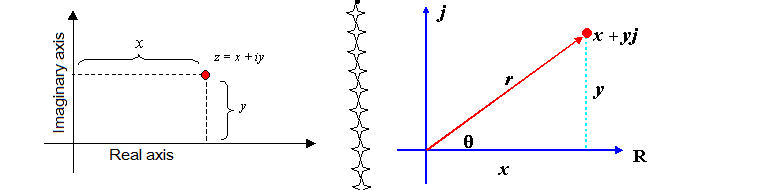$\boxed{\Large{ i= \sqrt{-1}}}$

• Every complex number $z$ will have a magnitude $|z|$ and an angle that it makes with the real axis, called it’s argument , denoted by $arg(z)$ and always $-\pi < arg(z) \leq \pi$ This is why we can treat complex numbers as vectors whenever required.

• If $z=x+iy$, then $|z|=\sqrt{x^2+y^2}$ and $arg(z) = tan^{-1}\frac{y}{x}$.

• A complex number of unit modulus making an angle $\theta$ with real axis will be given by $e^{i\theta}=\cos\theta+i\sin\theta \implies z = |z| e^{i\theta}$

• Argument of all positive real numbers is $0$ and that of all negative real numbers is $\pi$

$\quad\quad$ Argument of all numbers of the form $ki$ is $\frac{\pi}{2}$ for $k>0$ and $\frac{-\pi}{2}$ for $k<0$.

• Every complex number is equivalent to a vector from Origin (complex number 0) to the point where it is denoted. Thus $z= (z-0)$, a vector segment from $0$ to $z$.

• The complex number equivalent to the vector segment between $A$ and $B$ will be $\vec{AB}=b-a$ , and $\vec{BA}=a-b$.

• $arg(z_1z_2)=arg(z_1)+arg(z_2)$, adjust the $2\pi$ addition/subtraction to bring it in the range($-\pi,\pi$]

• $\dfrac{z_1}{z_2} = \dfrac{|z_1|}{|z_2|} \times e^{i\theta}$ , where $\theta$ is the angle to be traced from $z_2$ in anticlockwise direction, in order to reach the direction same as $z_1$. Thus in other words, $\theta = arg(z_1)-arg(z_2)$.

• As in above thing $|z_1|,|z_2|$ will be some real values, we can say $z_1 = k\times z_2 \times e^{i\theta}$ for all $z_1,z_2$ with $z_2\neq 0$.

$\quad\quad$ (Yeah, division by zero is not allowed in complex numbers too!)

$\quad\quad$ $k$ will be a real number which will help us adjust the magnitude of our complex number.

With this much machinery, we are equipped enough to obtain some results we’ll be actually needing in geometry problems, so let’s begin the actual bashing!

The notations will be standard, (G,H,O etc) except for $a,b,c$, which won’t be sides of triangles but the complex coordinates of the vertices $A,B,C$ respectively.

Here are $\color{#3D99F6}{\text{Some Results}}$ that will prove helpful,

(not dealing with their proofs, we want the ‘Use’ of them more than ‘Proof’)

$\boxed{1}$. Midpoint of segment between $z_1,z_2$ will be $\dfrac{z_1+z_2}{2}$

$\boxed{2}$. Centroid of a triangle with vertices $z_1,z_2,z_3$ will be $\dfrac{z_1+z_2+z_3}{3}$.

$\boxed{3}$. In a $\triangle ABC$, points $O,G,H$ are circumcenter, centroid, orthocenter respectively.
$\quad\quad$ As we know by Euler line, $2\vec{OG}=\vec{GH}$. If we take the complex numbers assigned to $O,G,H$ as $o,g,h$, then $2(g-o)=(h-g)$

$\therefore 2\left( \dfrac{a+b+c}{3} -o\right) = \left( h- \dfrac{a+b+c}{3} \right)$

$\quad\quad$ Now if we take the circumcircle of $\triangle ABC$ to be centered at origin, we get $2g=h-g \implies h=a+b+c$

In general, $h=a+b+c - 2o$, where $o$ is circumcenter coordinates.

$\boxed{4}$. If $\triangle ABC$ is equilateral, we have $|a-b|=|b-c|=|c-a|$ .

Also, if the names of vertices $A,B,C$ are in anticlockwise order, then $\vec{BA}$ is obtained by rotating $\vec{BC}$ by $60^\circ$ in anticlockwise direction,

$\therefore (a-b)=(c-b)e^{i\frac{\pi}{3}}$

$\boxed{5}$. If $AB \bot CD$, then you can say $(b-a)=k(c-d) i$ , where $k=\frac{|b-a|}{|c-d|}$ ,

Because $e^{i\frac{\pi}{2}}=\cos\frac{\pi}{2}+i\sin\frac{\pi}{2} = i$.

Now something interesting,

$\color{#3D99F6}{\text{Problems that use what’s discussed till now !}}$

Problems in this note are bit basic, but I feel you might feel them cool as the complex bashes are shorter than pure geometry... from next one there will be problems from Olympiads !

$\boxed{\mathbf{1}}$ Given any $\triangle ABC$, equilateral triangles are constructed externally on the sides of the triangle, to obtain points $P,Q,R$. Prove that centroid pf $\triangle PQR$ is same as centroid of $\triangle ABC$.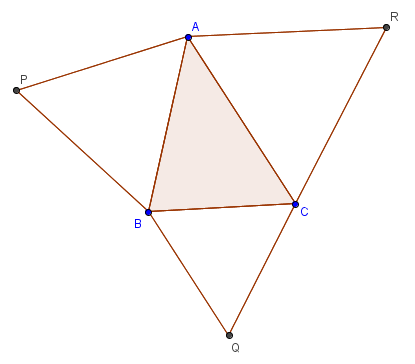$\textbf{Bash}$ – Let original triangle have complex coordinates $a,b,c$.

Now let’s use the simple fact that $PB$ is obtained by rotating $BA$ by $60^\circ=\frac{\pi}{3}$.

$\therefore (p-b)=(a-b)e^{i\frac{\pi}{3}} = (a-b)\left(\frac{1+\sqrt{3}i}{2}\right)$

$\therefore p=\frac{a+b}{2} + \frac{(a-b)\sqrt{3}i}{2}$

Similarly, $q= \frac{b+c}{2}+\frac{(b-c)\sqrt{3}i}{2}$ ; $r=\frac{c+a}{2}+\frac{(c-a)\sqrt{3}i}{2}$

Now centroid of $\triangle PQR = \frac{p+q+r}{3} = \dfrac{\frac{a+b}{2}+\frac{b+c}{2}+\frac{c+a}{2}+\frac{(a-b)\sqrt{3}i}{2}+\frac{(b-c)\sqrt{3}i}{2}+\frac{(c-a)\sqrt{3}i}{2}}{3} = \dfrac{a+b+c}{3}$

As centroid of $\triangle ABC$ is also $\dfrac{a+b+c}{3}$, we have proved the required result.

$\color{#D61F06}{\textbf{Note :-}}$ This will have solutions by pure geometry, but you can see they need more brain and are longer than what we did above….. But what’s more noteworthy is, imagine doing this by coordinate bash… You’d sink in the calculations to get points P,Q,R only!

$\boxed{\mathbf{2}}$ On the sides of $\triangle ABC$, squares are constructed externally ($\Box ABQP, \Box BCSR , \Box CAUT$). Prove that centroids of triangles $\triangle ABC , \triangle PRT , \triangle QSU$ are all the same point.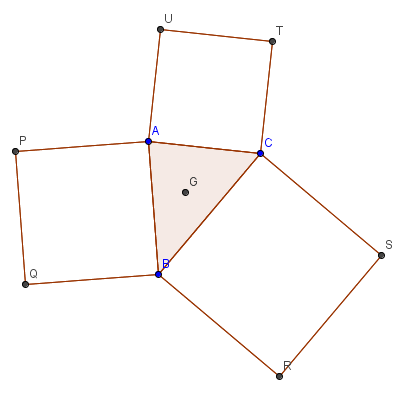$\textbf{Bash}$ – We use that $AP$ is rotated by $90^\circ = \frac{\pi}{2}$ in anticlockwise direction in order to get $AB$.

$\therefore (p-a) \times e^{i\frac{\pi}{2}} = (b-a) \\ \therefore (p-a)i = (b-a) \\ \therefore (p-a) i^2=(b-a)i\\ \therefore -p+a=(b-a)i \\ \therefore p=a+(a-b)i$

Now observe that $Q$ is just shift of $P$ by complex number $\vec{AB}=(b-a)$

$\therefore q=p+(b-a)=b+(a-b)i$

Similarly, we get all the required points, which are

$p=a+(a-b)i \\ q=b+(a-b)i \\ r=b+(b-c)i \\ s=c+(b-c)i \\ t= c+(c-a)i \\ u=a+(c-a)i$

Now it’s just easy calculation to find,

centroid of $\triangle PRT = \dfrac{ a+(a-b)i + b+(b-c)i + c+(c-a)i }{3}=\dfrac{a+b+c}{3}$

centroid of $\triangle QSU=\dfrac{ b+(a-b)i + c+(b-c)i + a+(c-a)i }{3} =\dfrac{a+b+c}{3}$

$\color{#D61F06}{\textbf{Note:-}}$ It’s noteworthy again that this solution is shorter and less brainy than Pure geometry and also, uses really very less calculations than Coordinate geometry…

With this, I’m concluding this part 2.

From next note onwards, there’ll be useful results and problems from past Olympiads.

If you enjoyed this, please share with your friends, who might be interested and seek help.

Stay tuned for more fun,

Happy Problem solving!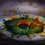6 years ago

This discussion board is a place to discuss our Daily Challenges and the math and science related to those challenges. Explanations are more than just a solution — they should explain the steps and thinking strategies that you used to obtain the solution. Comments should further the discussion of math and science.

When posting on Brilliant:

• Use the emojis to react to an explanation, whether you're congratulating a job well done , or just really confused .
• Ask specific questions about the challenge or the steps in somebody's explanation. Well-posed questions can add a lot to the discussion, but posting "I don't understand!" doesn't help anyone.
• Try to contribute something new to the discussion, whether it is an extension, generalization or other idea related to the challenge.

MarkdownAppears as
*italics* or _italics_ italics
**bold** or __bold__ bold
- bulleted- list
• bulleted
• list
1. numbered2. list
1. numbered
2. list
Note: you must add a full line of space before and after lists for them to show up correctly
paragraph 1paragraph 2

paragraph 1

paragraph 2

[example link](https://brilliant.org)example link
> This is a quote
This is a quote
    # I indented these lines
# 4 spaces, and now they show
# up as a code block.

print "hello world"
# I indented these lines
# 4 spaces, and now they show
# up as a code block.

print "hello world"
MathAppears as
Remember to wrap math in $$ ... $$ or $ ... $ to ensure proper formatting.
2 \times 3 $2 \times 3$
2^{34} $2^{34}$
a_{i-1} $a_{i-1}$
\frac{2}{3} $\frac{2}{3}$
\sqrt{2} $\sqrt{2}$
\sum_{i=1}^3 $\sum_{i=1}^3$
\sin \theta $\sin \theta$
\boxed{123} $\boxed{123}$

Sort by:

To @Parth Lohomi , thank you soooo much for that problem, saw it today and felt soooo happy, you remembered my brithday!

Want to see you too @IMOTC'16 :D

- 6 years ago

To the guys I'd like to meet in next year's IMOTC.... Something you'd like to know :)

@Satvik Golechha @Krishna Ar @Mehul Chaturvedi @Shivam Jadhav @Aditya Kumar @Harsh Shrivastava @Rishabh Tripathi

- 6 years ago

Hi! I'd love to meet you too one day; but that's surely not going to be IMOTC. In 10th my RMO went good but still I didn't get selected, and I ain't taking it in 11th (JEE fever :(

- 6 years ago

What! NO ! Please don't do that!

You can't just say 'JEE fever' and get your hands off Math Olympiads! If only you had been at the IMOTC, you'd know what it's like.... Dude take my word for this, the 1 month of IMOTC is 100 times more worth than 10 months of JEE coaching... Do NOT leave math Olympiad, even my teacher for JEE says that Olympiads never hamper the result of JEE, they'd enhance it....

This is for @Krishna Ar too, if you have any argument similar to @Satvik Golechha ....

- 6 years ago

Well, my argument is totally different (and pretty sane too!). It's just that I ain't that good.

- 6 years ago

Would really like to meet you . I am preparing for it . I got selected for INMO in class X but could not qualify it . Please help me with it. I have sent a mail to you regarding it . Please reply.

- 6 years ago

Well... dude.. I'd like to get some details on this from you. Can you talk to me on facebook please. ?

- 6 years ago

In which class are you sir!I too want to reach that height of IMOTC.I am in class 10th and haven't given RMO yet but I want to go for it,but how to prepare for it and also boards are on my head .I want to learn Mathematics through olympiads and (bashing as an alternative to problems).I always wanted to get advice from someone like you.

- 6 years ago

Haha! This note is just wonderful but :'( I am sure you are kidding me about the IMOTC part.

- 6 years ago

@Aditya Raut I'd love to have more notes on Geometry bashes. Thanks for the note! :)

- 6 years ago

Hi sir.I m in class 10 right now and preparing for rmo.I aspire to reach as high as you have gone but some people say that as I m in 10th I should focus more on ntse but I m more intrested in math olympiads and it seems difficult to me to prepare for both the olympiads simultaneosly.Please advice me on what to do.By the way,bash notes were great and helped me a lot.

- 3 years, 10 months ago

but how to find the intersection of 2 lines in complex bashing.

- 3 years, 10 months ago

Thank u very much ..it helped me a lot..

- 5 years, 3 months ago

hey! great work on the note. I would love to see it's next part. Maybe you can take up some problems from past olympiads, and some other useful results like reflections, and similarity. Anyways great work. :)

- 4 years, 10 months ago

Hi, is part 3 out? If not, please make one. Also, these notes are very useful, thank you.

- 3 months, 1 week ago